# Sketching Graphs Worksheet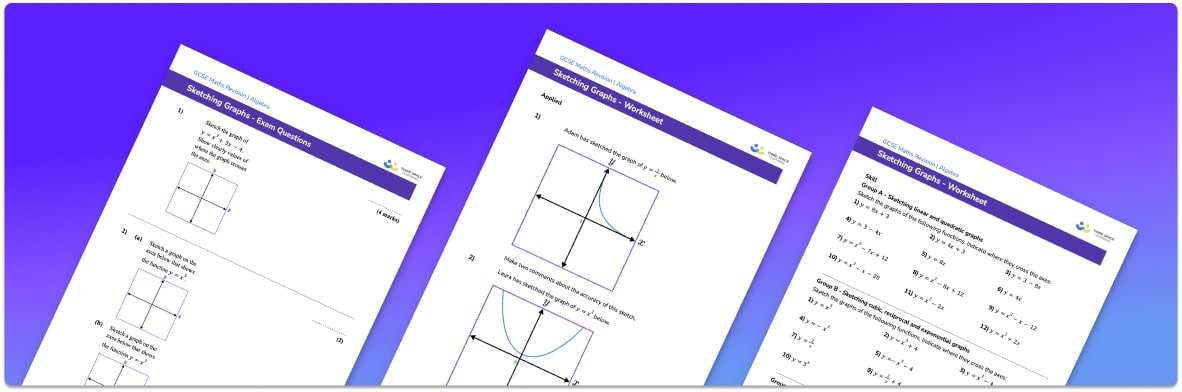• Section 1 of the sketching graphs worksheet contains 20+ skills-based sketching graphs questions, in 3 groups to support differentiation
• Section 2 contains 3 applied sketching graphs questions with a mix of worded problems and deeper problem solving questions
• Section 3 contains 3 foundation and higher level GCSE exam questions on sketching graphs
• Answers and a mark scheme for all sketching graphs questions are provided
• Questions follow variation theory with plenty of opportunities for students to work independently at their own level
• All questions created by fully qualified expert secondary maths teachers

Suitable for GCSE maths revision for AQA, OCR and Edexcel exam boards

• This field is for validation purposes and should be left unchanged.

You can unsubscribe at any time (each email we send will contain an easy way to unsubscribe). To find out more about how we use your data, see our privacy policy.

### Sketching graphs at a glance

In GCSE maths there are a number of different graphs that you need to know and be able to sketch. These include the following graphs: linear graphs, quadratic graphs, cubic graphs, reciprocal graphs, exponential graphs and trigonometric graphs.

When sketching graphs, we don’t need to accurately plot the graph and therefore we don’t label the axes. What we do do is mark on key points such as intersections with the x axis and y axis and coordinates of the turning points.

When sketching a graph, we first identify the shape of the graph. For example, a linear graph is a straight line and a quadratic graph is a parabola. We then identify the key points for that graph. For example, when sketching quadratic graphs, we can find the point of intersection with the y axis by substituting x=0 and finding y. We can then find the points of intersection with the x axis by setting y=0 and solving the quadratic equation. If the discriminant of the quadratic (b^{2}-4ac) is less than zero, we won’t be able to solve the equation meaning the graph does not intersect the x axis. Finally, we can complete the square to find the coordinates of the turning point of the quadratic function.

The exact process will vary depending on the type of graph we are being asked to sketch.

Looking forward, students can then progress to additional interpreting graphs worksheets and other algebra worksheets, for example a sequences worksheet, simultaneous equations worksheet or straight line graphs worksheet.For more teaching and learning support on Algebra our GCSE maths lessons provide step by step support for all GCSE maths concepts.

## Related worksheets

Inverse Functions Worksheet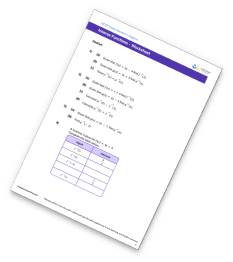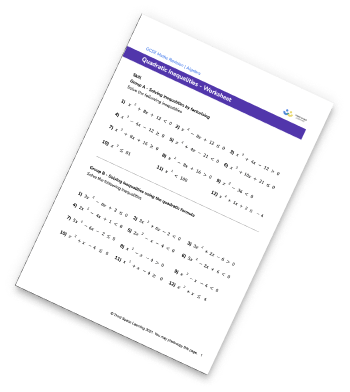Inequalities On A Number Line Worksheet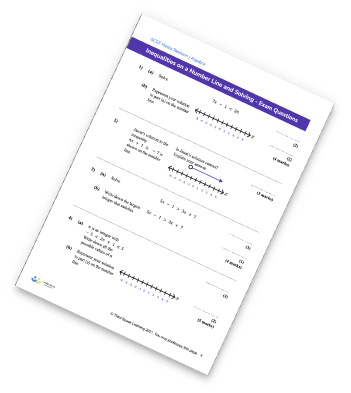Inequalities Worksheet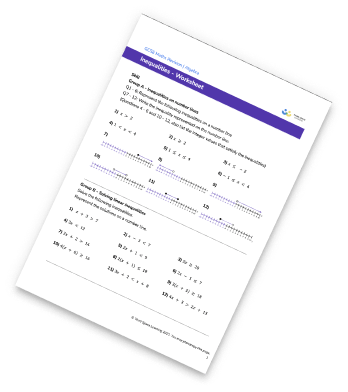## Do you have KS4 students who need more focused attention to succeed at GCSE?There will be students in your class who require individual attention to help them succeed in their maths GCSEs. In a class of 30, it’s not always easy to provide.

Help your students feel confident with exam-style questions and the strategies they’ll need to answer them correctly with our dedicated GCSE maths revision programme.

Lessons are selected to provide support where each student needs it most, and specially-trained GCSE maths tutors adapt the pitch and pace of each lesson. This ensures a personalised revision programme that raises grades and boosts confidence.

Find out more